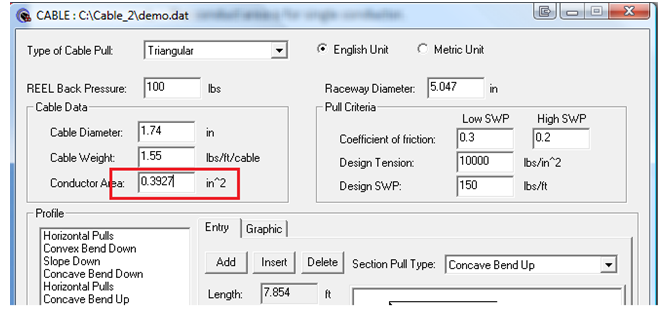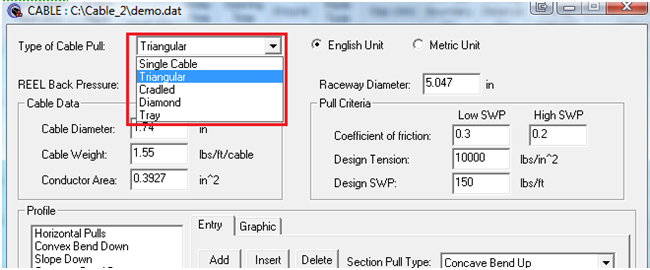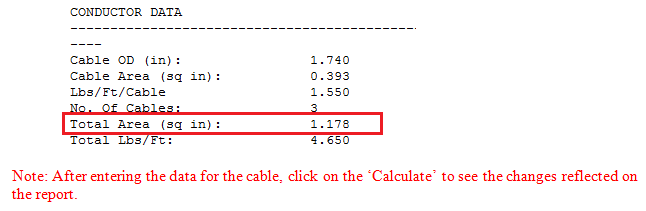# Calculating the Total Conductor Area

Follow the following steps to calculate the total Conductor Area in Cable 3D

1. The conductor area is for single conductor2. The “Type of Cable Pull” decides the number of the conductors. Triangular refers to 3 conductor while Diamond refers to 4 conductors.3. The "Total Area" is the conductor area.